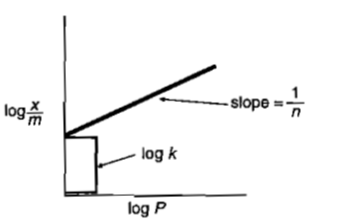# NEET Chemistry Surface Chemistry Questions Solved

NEET - 2006

A plot of log x/m verses log p for the adsorption of a gas on a solid gives a straight line with slope equal to:-

(a) -log k
(b) n
(c) 1/n
(d) log k

The empirical relation x/m= k p1/n, put forward by Freundlich is known as Freundlich adsorption isotherm.Taking logarithm
log x/m=log k+1/n log p

If a following curve is plottedDifficulty Level:

• 7%
• 5%
• 78%
• 12%